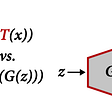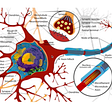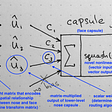# Backpropagation Deep Dive

## Back Propagation with Softmax output and CrossEntropy Loss

The internet is teeming with articles explaining Backpropagation. Why one more? Well, I set out to understand this and thought I understood this, having derived out one and done a toy example too. But then I tried to code a simple CNN in python and immediately many things started falling apart.

There are different levels of understanding here. For many DeepLearning applications, it is enough to understand at a higher level the backpropagation and other algorithms. Let’s try to go a bit deeper.

Note -Medium does not support MathJax, and the article is chock-a-block with MathJax. Wherever inline formulas are there I am writing this out like a_i where i is the subscript or w^l where l is the superscript. This is needed only in a few places. Hence here is the direct link to the same if you need to read this clearly https://alexcpn.github.io/html/NN/ml/7_cnn_network/.

## The Maths you Need for Back Propagation and the Maths you probably don’t

There is a vast amount of articles and explanations, I will be referring mostly to a few root references here.

• Understand what Scalar’s, Vectors, Tensors are and that Vectors and Tensors are written as matrices and Vector is one dimension matrix whereas Tensor’s are many dimensional usually. (Technically a Vector is also a Tensor). After this, you can forget about Tensors and think only of Vectors Matrices and Scalar. Mostly just matrices.
• That linear algebra for matrices that will be used is just properties of matrix multiplication and addition that you already know. A linear equation of the form y=m∗x+c in matrix form used in a neural network is
• The Index notation for dealing with Vectors and MatricesA Primer on Index Notation John Crimaldi
• Matrix multiplication plays a major part and there are some parts that may be confusing
• Example- Dot Product is defined only between Vectors, though many articles and tutorials will be using the dot product. Since each row of a multidimensional matrix acts like a Vector, the Numpy dot function(numpy.dot) works for matrix multiplication for non-vectors as well. Technically numpy matmul is the right one to use for matrix multiplication. np.dot(A,B) is same as np.matmul(A,B).numpy.Numpy einsum is also used for dimensions more than two. If A and B are two dimensional matrices np.dot(A,B)=np.einsum(‘ij,jk−>ik′,A,B). And einsum is much easier than numpy.tensordot to work with. For Hadamard product numpy.multiply
• There is no accepted definition of matrix multiplication of dimensions higher than two!
• Hadamard product. It is a special case of the element-wise multiplication of matrices of the same dimension. It is used in the magic of converting index notation to Matrix notation. You can survive without it, but you cannot convert to Matrix notation without understanding how. It is referred to in Michel Neilsen's famous book Neural Networks and Deep Learning Michel Neilsen in writing out the Error of a layer with respect to previous layers.
• Calculus, the concept of Derivatives, Partial Derivatives, Gradient, Matrix Calculus, Jacobian Matrix
• That derivative of a function -the derivative function f′(x), gives the slope or gradient of the function at any ‘point’. As it is the rate of change of one variable with respect to another. Visually, say for a function in 2D space, say a function representing a line segment, that means a change in Y for a change in X — rise over run, slope.
• For multivariable function, for example, a Vector function, we need the rate of change of many variables with respect to another, we do so via Partial derivatives concept-notation ∂ , and the gradient becomes a Vector of partial derivatives. To visualize this, picture a hill, or a function of x,y,z variables that can be plotted in a 3D space, a ball dropped on this hill or graph goes down this gradient vector .To get the derivative function f′(x,y,z) to calculate this gradient you need, again something that you can ignore most of the time, except the slightly different rules while calculating the derivative function.
• Take this a notch further and we reach the Jacobian matrix. For a Vector of/containing multivariable functions, the partial derivatives with respect to say a Matrix or Vector of another function gives a Matrix of Partial Derivatives called the Jacobian Matrix. And this is also a gradient matrix. It shows the ‘slope’ of the derivative function at a matrix of points. In our case the derivative of the Loss function (which is a scalar function) with respect to Weights (matrix), can be calculated only via intermediate terms, that include the derivative of the Softmax output (Vector) with respect to inputs (matrix) which is the Jacobian matrices. And that is matrix calculus. Again something that you can now ignore henceforth.
• Knowing what a Jacobian is, and how it is calculated, you can blindly ignore it henceforth. The reason is that most of the terms of the Jacobian evaluate to Zero for Deep learning application, and usually, only the diagonal elements hold up, something which can be represented by index notation. “So it’s entirely possible to compute the derivative of the softmax layer without actual Jacobian matrix multiplication …the Jacobian of the fully-connected layer is sparse.- The Softmax function and its derivative-Eli Bendersky
• Note -When you convert from Index notation to actual matrix notation, for example for implementation then you will need to understand how the index multiplication transforms to Matrix multiplication — transpose. Example from The Matrix Calculus You Need For Deep Learning (Derivative wrto Bias) Terence,Jermy

Calculus — Chain Rule — Single variable, Multivariable Chain rule, Vector Chain Rule

• The chain rule is used heavily to break down the partial derivate of the Loss function with respect to weight into a chain of easily differentiable intermediate terms
• The Chain rule that is used is actually Vector Chain Rule, but due to the nature of Jacobian matrices generated- sparse matrices, this reduces to resemble Chain rule of a single variable or Multi-variable Chain Rule. Again the definite article to follow is The Matrix Calculus You Need For Deep Learning (Derivative wrto Bias) Terence,Jermy, as some authors refer as Multivariable Chain rule in their articles

Vector Chain Rule

In the notation below, y is a Vector output and x is a scalar. Vectors are represented in bold letters though I have skipped it here.

Here both the terms on the RHS are two Jacobian matrices containing the set of partial derivatives. But since only the diagonals remain in deep learning application we can skip calculating the Jacobian and write as

# The Neural Network Model

I am writing this out, without index notation, and with the superscript representing just the layers of the network.

YY is the target vector or the Truth vector. This is a one-hot encoded vector, example Y=[0,1,0]Y=[0,1,0], where the second element is the desired class.The training is done so that the CrossEntropyLoss is minimised using the Gradient Loss algorithm.

P is the Softmax output and is the activation of the last layer a^l. This is a vector. All elements of the Softmax output add to 1; hence this is a probability distribution, unlike a Sigmoid output. The Cross-Entropy Loss LL is a Scalar.

Note the Index notation is the representation of an element of a Vector or a Tensor and is easier to deal with while deriving out the equations.

Softmax (in Index notation)

Below I am skipping the superscript part, which I used to represent the layers of the network.

This represents one element of the softmax vector, example

Cross-Entropy Loss (in Index notation)

Here y is the indexed notation of an element in the target vector Y.

# On to the rest of the explanation

There are too many articles related to Backpropagation, many of which are very good. However many explain in terms of index notation and though it is illuminating, to really use this with code, you need to understand how it translates to Matrix notation via Matrix Calculus and with help from StackOverflow related sites.

## CrossEntropy Loss with respect to Weight in the last layer

If you are confused with the indexes, just take a short example and substitute. Basically, i,j,k etc are dummy indices used to illustrate in index notation the vectors.

I am going to drop the superscript l denoting the layer number henceforth and focus on the index notation for the softmax vector PP and target vector YY

Repeating from Derivative of Softmax Activation -Alijah Ahmed

The last term is the derivative of Softmax with respect to its inputs also called logits. This is easy to derive and there are many sites that describe it. Example Derivative of SoftMax Antoni Parellada. The more rigorous derivative via the Jacobian matrix is here The Softmax function and its derivative-Eli Bendersky

Using this above and repeating from Derivative of Softmax Activation -Alijah Ahmed

these i and j are dummy indices and we can rewrite this as

taking the two cases and adding in the above equation

We need to put this back in 1EqA1. We now need to calculate the second term, to complete the equation

Putting all together

Now let’s do the derivation for the inner layers

# Derivative of Loss with respect to Weight in Inner Layers

The trick here (yes it is a trick), is to derive the Loss with respect to the inner layer as a composition of the partial derivative we computed earlier. And also to compose each partial derivative as a partial derivative with respect to either z_x or w_x but not with respect to a_x. This is to make derivatives easier and intuitive to compute.

The trick is to represent the first part in terms of what we computed earlier

Note — Value of EqA2.1 to be used in the next layer derivations; and repeated to the first layer; ie do not repeat (p_i -y_i)

Disclaimer As proud I am to understand till here, I had the misfortune of trying to implement a CNN with this and I know what is all wrong with the above. Basically, if you use the above equation, you will find that the weights do not match for matrix multiplication.

This is because the above equation is correct only as far as the index notation is concerned. But practically we work with weight matrices, and for that, we need to write this Equation in Matrix Notation. For that, some of the terms become Transposes, some matrix multiplication (dot product style) and some Hadamard product. (⊙). All these are detailed out in The Matrix Calculus You Need For Deep Learning Terence,Jermy, and I need to edit the answer and explanation once I have grasped it with an example. Only then will the weight dimension align correctly. Please see the correct equations here Neural Networks and Deep Learning Michel Neilsen

One example

Update

The transformation to Hadamard product from index notation, I finally got, and it is here https://stats.stackexchange.com/questions/566916/jacobian-matrix-of-an-element-wise-operation-on-a-matrix. When I get time I will update this.

# References

Easier to follow (without explicit Matrix Calculus) though not really correct

Easy to follow but lacking in some aspects

Slightly hard to follow using the Jacobian

More difficult to follow with proper index notations (I could not) and probably correct

Some of the other references

[A Primer on Index Notation John Crimaldi]: https://web.iitd.ac.in/~pmvs/courses/mcl702/notation.pdf

[The Matrix Calculus You Need For Deep Learning Terence,Jermy]:https://arxiv.org/pdf/1802.01528.pdf

[The Matrix Calculus You Need For Deep Learning (Derivative with respect to Bias) Terence,Jermy]: https://explained.ai/matrix-calculus/#sec6.2

[Neural Networks and Deep Learning Michel Neilsen]: http://neuralnetworksanddeeplearning.com/chap2.html

[lecun-ranzato]: https://cs.nyu.edu/~yann/talks/lecun-ranzato-icml2013.pdf

[The Softmax function and its derivative-Eli Bendersky]: https://eli.thegreenplace.net/2016/the-softmax-function-and-its-derivative/

[Python Implementation of Jacobian of Softmax with respect to Logits Aerin Kim]: https://stackoverflow.com/a/46028029/429476

[Derivative of Softmax Activation -Alijah Ahmed]: https://math.stackexchange.com/questions/945871/derivative-of-softmax-loss-function

[Dertivative of SoftMax Antoni Parellada]: https://stats.stackexchange.com/a/267988/191675

[Backpropagation In Convolutional Neural Networks Jefkine]: https://www.jefkine.com/general/2016/09/05/backpropagation-in-convolutional-neural-networks/]

[Finding the Cost Function of Neural Networks Chi-Feng Wang]: https://towardsdatascience.com/step-by-step-the-math-behind-neural-networks-490dc1f3cfd9

--

--

--

## More from Better ML

Software Engineering for ML  — Implementation, Versoning, Operations

## VEHICLE WARNING INDICATOR SYSTEM## How Machine Learning & Augmented Reality Crushed It at Google I/O 2018## Detecting Trustworthy Domains## Generative Adversarial Networks and Variants## Data-Efficient GANs!## Feature Selection For Dimensionality Reduction(Wrapper Method)## Machine Learning Pipelines: Everything You Need to Know## Alex Punnen

SW Architect/programmer- in various languages and technologies from 2001 to now. https://www.linkedin.com/in/alexpunnen/

## Perceptron: Simplified## Good Sources to Learn CNNs, CapsNet, ViT, and GNNs — 2## Detecting Malaria with Deep Learning